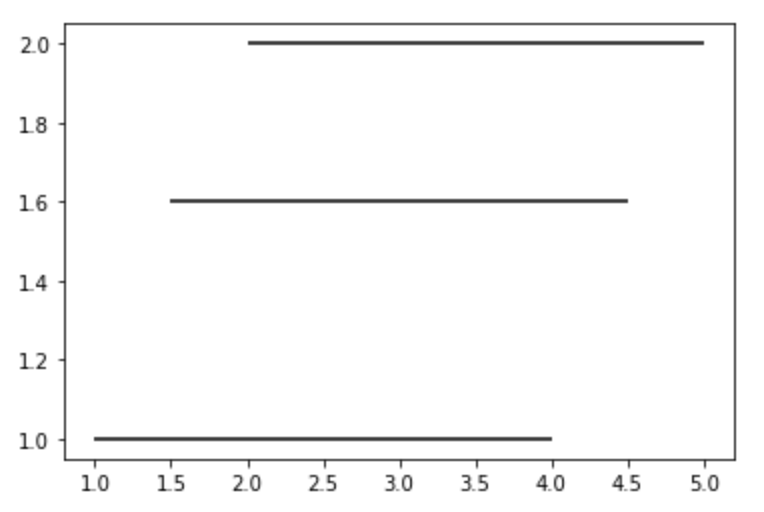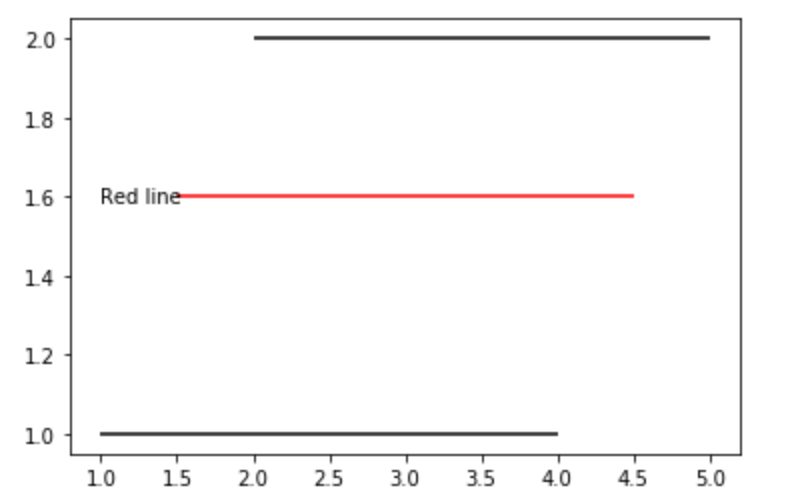# Matplotlib.pyplot.hlines() in Python

• Last Updated : 19 Apr, 2020

Matplotlib is an amazing visualization library in Python for 2D plots of arrays. Matplotlib is a multi-platform data visualization library built on NumPy arrays and designed to work with the broader SciPy stack.

## Matplotlib.pyplot.hlines()

The `Matplotlib.pyplot.hlines()` is used to draw horizontal lines in a graph at each y from xmin to xmax.

Attention geek! Strengthen your foundations with the Python Programming Foundation Course and learn the basics.

To begin with, your interview preparations Enhance your Data Structures concepts with the Python DS Course. And to begin with your Machine Learning Journey, join the Machine Learning - Basic Level Course

Syntax: matplotlib.pyplot.hlines(y, xmin, xmax, colors=’k’, linestyles=’solid’, label=”, *, data=None, **kwargs

Parameters:
The `Matplotlib.pyplot.hlines()` accepts the below-described parameters:

• y : It is a required parameter for this method. This parameter describes that in the graph the line is to be drawn. Its value is a scalar or sequence of scalars, in other words, it is the y-indexes where the line is to be plotted.
• xmin: It is a required parameter that has either a scalar value or a 1D array-like value that sets the beginning of each line. If scalars are provided all the lines will have the same length.
• colors: As the name suggests it is used to set the color of the line to be plotted. This parameter is optional in nature and its default value is ‘k’
• linestyles: It is also an optional parameter that accepts four values namely ‘solid’, ‘dashed’, ‘dashdot’ and ‘dotted’. It is responsible for setting the style of the line to be plotted.
• label: It is an optional parameter used to describe information about the plotted line in the same line. This accepts a string whose default value is an empty string.
• **kwargs: This parameter is used to make use of LineCollection properties in the plotted line.

Note: In addition to the above-mentioned parameters, this method can take a data keyword argument. It is also important to note that the object passed as data must support item access and membership test.

Example 1:
 `from` `matplotlib ``import` `pyplot as plt`` ` `plt.hlines(y ``=` `1``, xmin ``=` `1``, xmax ``=` `4``)`` ` `plt.hlines(y ``=` `1.6``, xmin ``=` `1.5``, xmax ``=` `4.5``)`` ` `plt.hlines(y ``=` `2``, xmin ``=` `2``, xmax ``=` `5``)`

Output :Example 2:

 `from` `matplotlib ``import` `pyplot as plt`` ` `plt.hlines(y ``=` `1``, xmin ``=` `1``, xmax ``=` `4``, label ``=``"black line"``)`` ` `plt.hlines(y ``=` `1.6``, xmin ``=` `1.5``, xmax ``=` `4.5``, color ``=``'r'``)``plt.text(``1``, ``1.6``, ``'Red line'``, ha ``=``'left'``, va ``=``'center'``)`` ` `plt.hlines(y ``=` `2``, xmin ``=` `2``, xmax ``=` `5``)`

Output :My Personal Notes arrow_drop_up